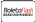Capa do livro de Pentadiagonal Matrix
Título do livro:

## Linear Algebra, Matrix (Mathematics), Diagonal Matrix

Tract (2011-11-11 )Apto para vale
ISBN- 1 3:

### 978-613-8-58980-8

ISBN- 1 0:
6138589807
EAN:
9786138589808
Idioma do livro:
Inglês
Anotações e citações/ texto breve:
In linear algebra, a pentadiagonal matrix is a matrix that is nearly diagonal; to be exact, it is a matrix in which the only nonzero entries are on the main diagonal, and the first two diagonals above and below it. So it is of the formIt follows that a pentadiagonal matrix has at most 5n − 6 nonzero entries, where n is the size of the matrix. Hence, pentadiagonal matrices are sparse. This makes them useful in numerical analysis.
Editora:
Tract
Website:
http://www.betascript-publishing.com
Eloi Rylan Koios
Número de páginas:
72
2011-11-11
Stock:
Disponível
Categoria:
Ciências Naturais Gerais
Preço:
187.92 R\$
Palavras chave:
Linear Algebra, Matrix (mathematics), Diagonal Matrix### Categorias